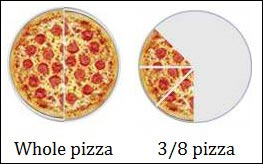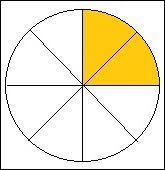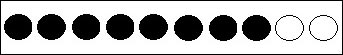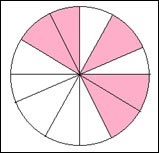# Introduction

#### Complete Python Prime Pack

9 Courses     2 eBooks

#### Artificial Intelligence & Machine Learning Prime Pack

6 Courses     1 eBooks

#### Java Prime Pack

9 Courses     2 eBooks

A fraction is a part of a whole.For example, a pizza is sliced into eight equal parts and we represent 3 parts of the pizza as a fraction 3/8

The top number is called the numerator and the bottom number is called the denominator.

The numerator gives the number of slices we have (here 3) and the denominator gives the number of slices the pizza is cut into (here 8).

The circle below is cut in 8 equal slices. What fraction of the circle is shaded?### Solution

Step 1:

We see that 2 of the 8 slices are shaded. We write this as a fraction

Number of shaded slices/Total number of slices = 2/8

Step 2:

So the answer is 2/8

The numerator is 2 and the denominator is 8

Jack has ten marbles. What fraction of his marbles are white?### Solution

Step 1:

Number of marbles that are white is 2

The total number of marbles 10

Step 2:

So the fraction of marbles that are white is 2/10

The figure below is cut into 12 equal slices. What fraction of the figure is shaded?### Solution

Step 1:

Number of pieces shaded is 5 and the total number of pieces is 12.

Step 2:

So, the fraction of the shaded pieces is 5/12.